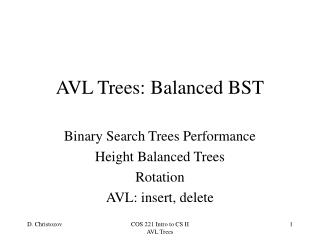DownloadDownload PresentationAVL Trees: Balanced BST

# AVL Trees: Balanced BST

Download Presentation## AVL Trees: Balanced BST

- - - - - - - - - - - - - - - - - - - - - - - - - - - E N D - - - - - - - - - - - - - - - - - - - - - - - - - - -
##### Presentation Transcript

1. AVL Trees: Balanced BST Binary Search Trees Performance Height Balanced Trees Rotation AVL: insert, delete COS 221 Intro to CS II AVL Trees

2. Binary Search Trees: Performance The number of operations in function ‘search’ is equal to the length of the path from the root to the leaf. Therefore the worst case of search for (unbalanced) BST is O(n) The worst case of balanced BST is O(log2n) COS 221 Intro to CS II AVL Trees

3. n-1 n Height-balanced trees A height-balanced binary tree has the property thatfor each node the difference in the heights of the right and left child is no larger than one. Full and Complete binary tree are height-balanced tree. COS 221 Intro to CS II AVL Trees

4. Height-balanced trees Theorem: The paths in height-balanced binary trees have logarithmic length. The largest number of nodes in a binary tree with n levels (of depth n) is 2n-1. Let us denote with Mn the minimal number of nodes in height-balanced tree of depth n. M0 = 1M1 = 2Mn+1 = Mn-1 + Mn + 1 Similar to Fibonacci numbers: fn+1 = fn-1 + fn and thereforewe can approximate Mn and n≈ 1.44 log2 Mn COS 221 Intro to CS II AVL Trees

5. Let only for the node A the height-balanced tree property is violated and its depth is n+1. Let the right child B is heavier – its depth is n. The depth of X is n-2; and the depth of Y and Z are either n-1 or n-2. We can assume that rotation to left will restore the height-balance property of the tree. Left rotation is performed with two assignments: A->right(B->left); B->left(&A) A B X X Y Y Z Z A B Algorithms to maintain the Height-Balance Property in a Binary Search Tree is based on Rotation Rotation Before Rotation: After Rotation: COS 221 Intro to CS II AVL Trees

6. A Bbf = {0, 1, -1} Abf = 2 B X X Y Y Z Z Left rotation A B Rotation Heights before rotation Heights after rotation Bbf = (n-2) - n =-2 COS 221 Intro to CS II AVL Trees

7. AVL tree node: bf – balance factor Value = {Key, Data} int bf left right • Adelson-Velskii and Landis AVL tree: • The AVL tree is a Binary Search Tree • The AVL tree is a Height-Balanced Tree AVL Tree • The bf = {-1, 0, 1} • for every node in the tree • If bf > 1 and the bf of the root of the right-sub-tree is >=0 single left rotation restores the balance. • If bf > 1 and the bf of the root of the right-sub-tree is <0 to restore the balance double rotation is required: • single right rotation for the right sub-tree root; and • single left rotation for the current node. • for bf <-1 operation are symmetrical. COS 221 Intro to CS II AVL Trees

8. 9:0 15:0 5:0 12:-1 18:0 3:0 7:1 2:0 4:0 8:0 10:0 16:0 20:0 12:-2 new 11:0 11:? 10:1 AVL tree: insertion if(left->balFac != oldbf && left->balFac) balFac--; Update of balance factors follows the way back of the path of insertion. Insertion of the new node as right child increments the balance factor Unbalanced: Double rotation COS 221 Intro to CS II AVL Trees

9. 11:0 12:-2 2 10:1 12:-2 12:0 12:0 10:0 1 11:0 11:-1 10:0 AVL tree: insertion Direction of rotation depends on the sign of the balance factor: positive  left rotation; negative  right rotation. 12:-2 Double rotation: 1: Rotation around the left child Double rotation: 2: Rotation around the unbalanced node. COS 221 Intro to CS II AVL Trees

10. Further update of balance factors follows the way back of the path of node removal. (the left-most descendent) 9:1 15.8:-1 15:1 5:-1 if(left->balFac != oldbf && left->balFac == 0) balFac--; 18:-1 16:-1 20:1 7:1 17 25 8:0 15.9:0 11:-1 3:1 10:-1 12:0 2:0 4:-1 11.5:0 3.5 • Follows the algorithm for removal in a BST • Updates the balance factors • Restores balance, when needed AVL tree: removal Let us remove 15 9:0 15.8:0 The parent’s balance factor is incremented: the left sub-tree has one level less 18:0 16:0 15.9:0 It’s right child will become the left child of its parent. The left-most descendent of the right sub-tree is 15.8 COS 221 Intro to CS II AVL Trees